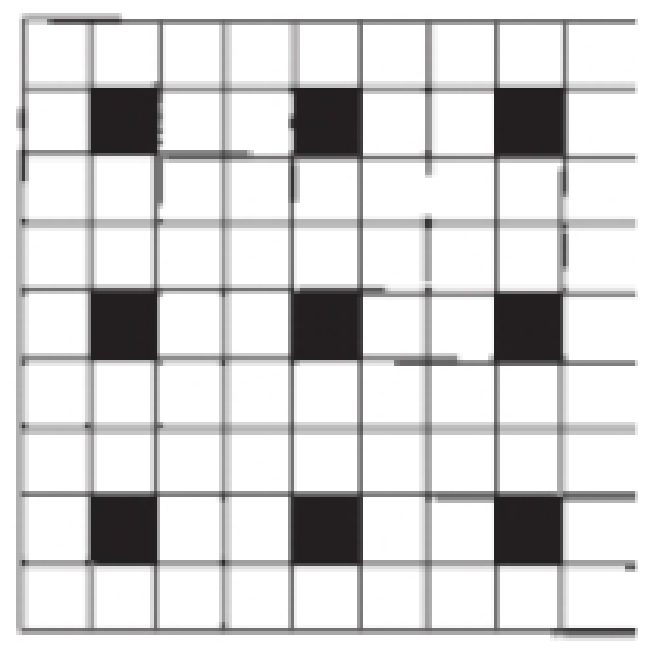# Outline a two-dimensional unit cell for the pattern shown here. If the black squares are labeled A and the white squares are B, what is the simplest formula for a “compound” based on this pattern?BuyFind

### Chemistry & Chemical Reactivity

10th Edition
John C. Kotz + 3 others
Publisher: Cengage Learning
ISBN: 9781337399074BuyFind

### Chemistry & Chemical Reactivity

10th Edition
John C. Kotz + 3 others
Publisher: Cengage Learning
ISBN: 9781337399074

#### Solutions

Chapter 12, Problem 1PS
Textbook Problem

## Outline a two-dimensional unit cell for the pattern shown here. If the black squares are labeled A and the white squares are B, what is the simplest formula for a “compound” based on this pattern?Expert Solution
Interpretation Introduction

Interpretation:

The simplest formula of the compound has to be identified.

## Answer to Problem 1PS

The simplest formula of the compound is AB8.

### Explanation of Solution

According to the picture, we consider the unit pattern as combination of two molecules,

There are seventy two white squares and nine black squares in the two dimensional unit cell patterns.

Formulaofthepattern=9black squares+72white squares+therefore,Formulaofthepattern=A9(blacksquares)+B72(white  squares)SimplfythepatternSimplestformulaofthepattern=AB8

Conclusion

The simplest formula of the compound was identified.

### Want to see more full solutions like this?

Subscribe now to access step-by-step solutions to millions of textbook problems written by subject matter experts!

### Want to see more full solutions like this?

Subscribe now to access step-by-step solutions to millions of textbook problems written by subject matter experts!© 2021 bartleby. All Rights Reserved.